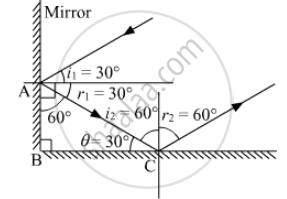Advertisement Remove all ads

# Draw a Figure Describing the Following. The reflecting surface of two mirrors make an angle of 90∘ with each other. If a ray incident of one mirror has an angle of incidents of 30∘ - Science

Diagram
Sum

Draw a figure describing the following.

The reflecting surface of two mirrors make an angle of 90 with each other. If a ray incident of one mirror has an angle of incidents of 30, draw the ray reflected from the second mirror. What will be its angle of reflection?

Advertisement Remove all ads

#### Solution

Let r2 be the angle of reflection from the second mirror.From laws of reflection,

angle i _1 = angle r_1 = 30°

Now in triangle ABC

θ = 180° - 90° - 60° = 30°

⇒ i2 = 60°

Hence , r2 = 60°

The angle of reflection of incident ray is 60.

Concept: Reflection of Light
Is there an error in this question or solution?
Advertisement Remove all ads

#### APPEARS IN

Balbharati General Science 8th Standard Maharashtra State Board
Chapter 16 Reflection of Light
Exercise | Q 2 | Page 115
Advertisement Remove all ads
Advertisement Remove all ads
Share
Notifications

View all notifications

Forgot password?